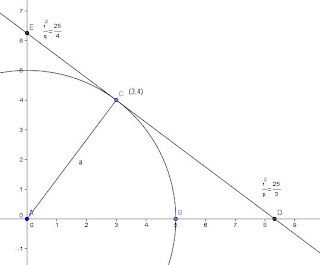## Tuesday, 10 November 2009

### Why Didn't I know This Already?I was playing with a problem that Dave Renfro sent to the AP Calculus EDG and got curious about something else, and stumbled upon a simple relationship about tangents to circles that I never knew (or don't remember??? )

The problem Dave sent was about finding the common tangent to a circle centered on the origin and a parabola with a vertex at the origin. I wandered over to playing around with a general solution, and along the way I remembered a cool idea that was known way back to Apollonius, I think. If you have a parabola centered at the origin, like y=ax2, and you draw a tangent to some point, call it (p,q), then the y-intercept of that tangent line will be at (0,-q)... no matter what the value of a is. And it seems clear that a straight line that goes from (p,q) to (0,-q) must have an x-intercept at (p/2,0)

For example, if you used y=x2 at the point (2,4) the tangent would have an equation of y-4 = 4(x-2) and when x= 0, the y-intercept is at (0,-4). If instead you pick y= 3x2, when x=2, y= 12. The equation of the tangent will by y-12 = 12(x-2) and the y-intercept will be at (0,-12), the x-intercept at (1,0)in both cases.

It occurred to me that probably all the conics might have simple relationships that would predict intercepts of the tangent. So I started with a circle.... say x2+y2= r2. What happens if we pick a point (p,q)? As I wrote in a recent blog, an easy way to find the tangent to a conic is by the use of polars. If we want the tangent to a circle at a point (p,q) we need only to replace one x and one y in the equation with the values p and q. In the case of x2+y2= r2, the tangent through the point (p,q) would be px+qy=r2. Pretty easy (so why had I played with this all these years and never noticed that the x-intercept would be r2/p, and the y-intercept would be r2/q. So why had I never noticed this relationship??? (don't answer, the truth hurts)

So a simple hyperbola or ellipse could be done the same way... tooooo easy...